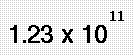_ N_ Scientific Notation
 • Introductory Comments • What is Molecular Modeling? • Why is Molecular Modeling Important? • What do some common molecules look like? • Where's the Math? • Carbon 3 Ways • Carbon Compounds • Water and Ice • Water and Ice pt.II • How to view structures in class or at home • MathMol Library of Structures.• Tutorial 1: 1-Dimension, 2-Dimensions, 3-Dimensions... • Tutorial2: The Geometry of 2 Dimensions.. • Tutorial3: The Geometry of 3- Dimensions • Tutorial4: The Geometry of Molecules. • Appendix1: Scientific Notation • Appendix 2: Mass • Appendix3: Volume • Appendix4: Density

Do you know this number, 300,000,000 m/sec.?

It's the Speed of light !

Do you recognize this number, 0.000 000 000 753 kg. ?

This is the mass of a dust particle!

Scientists have developed a shorter method to express very large numbers. This method is called scientific notation. Scientific Notation is based on powers of the base number 10.

The number 123,000,000,000 in scientific notation is written as :The first number 1.23 is called the coefficient. It must be greater than or equal to 1 and less than 10.

The second number is called the base . It must always be 10 in scientific notation. The base number 10 is always written in exponent form. In the number 1.23 x 1011 the number 11 is referred to as the exponent or power of ten.

### To write a number in scientific notation:

Put the decimal after the first digit and drop the zeroes.In the number 123,000,000,000 The coefficient will be 1.23

To find the exponent count the number of places from the decimal to the end of the number.

In 123,000,000,000 there are 11 places. Therefore we write 123,000,000,000 as:Exponents are often expressed using other notations. The number 123,000,000,000 can also be written as:

1.23E+11 or as 1.23 X 10^11

For small numbers we use a similar approach. Numbers less smaller than 1 will have a negative exponent. A millionth of a second is:

0.000001 sec. or 1.0E-6 or 1.0^-6 or### Try These sample questions!

Type the number for each in the box provided then hit the submit button.
5.3 X 10-3=

6.34 X 105=

5.56 X 107=